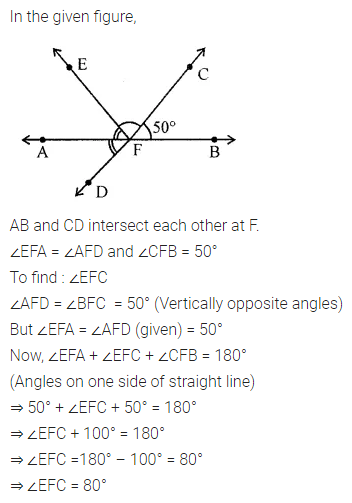ML Aggarwal Class 7 Solutions Chapter 10 Lines and Angles Ex 10.1 for ICSE Understanding Mathematics acts as the best resource during your learning and helps you score well in your exams.

## ML Aggarwal Class 7 Solutions for ICSE Maths Chapter 10 Lines and Angles Ex 10.1

Question 1.
(i) Can two right angles be complementary?
(ii) Can two right angles be supplementary?
(iii) Can two adjacent angles be complementary?
(iv) Can two adjacent angles be supplementary?
(v) Can two obtuse angles be adjacent?
(vi) Can an acute angle be adjacent to an obtuse angle?
(vii) Can two right angles form a linear pair?
Solution: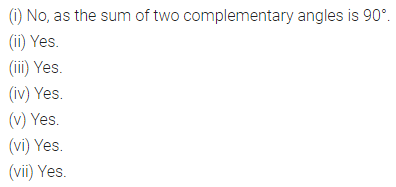Question 2.
Find the complement of each of the following angles: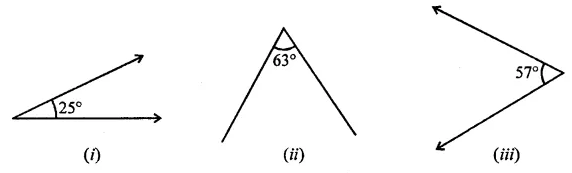Solution: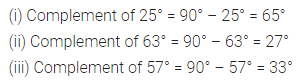Question 3.
Find the supplement of each of the following angles: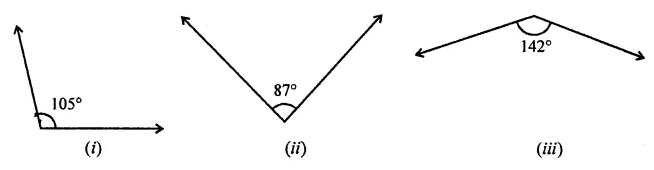Solution:Question 4.
Identify which of the following pairs of angles are complementary and which are supplementary:
(i) 55°, 125°
(ii) 34°, 56°
(iii) 137°, 43°
(iv) 112°, 68°
(v) 45°, 45°
(vi) 72°, 18°
Solution: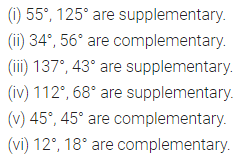Question 5.
(i) Find the angle which is equal to its complement.
(ii) Find the angle which is equal to its supplement.
Solution: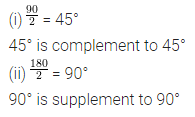Question 6.
Two complementary angles are (x + 4)° and (2x – 7)°, find the value of x.
Solution:Question 7.
Two supplementary angles are in the ratio of 2 : 7, find the angles.
Solution:Question 8.
Among two supplementary angles, the measure of the longer angle is 44° more than the measure of the smaller angle. Find their measures.
Solution: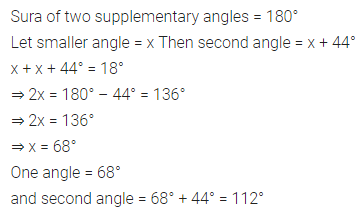Question 9.
If an angle is half of its complement, find the measure of angles.
Solution:Question 10.
Two adjacent angles are in the ratio 5 : 3 and they together form an angle of 128°, find these angles.
Solution: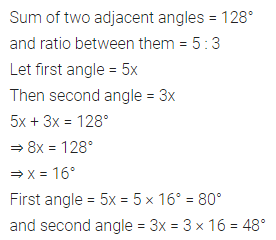Question 11.
Find the value of x in each of the following diagrams: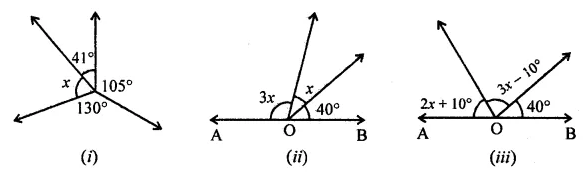Solution: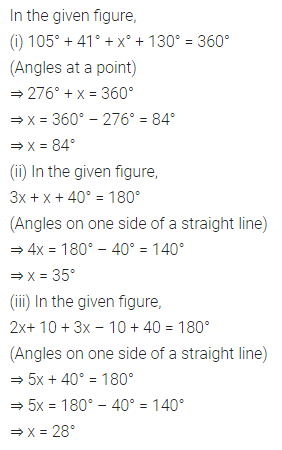Question 12.
Find the values of x, y and z in each of the following diagrams: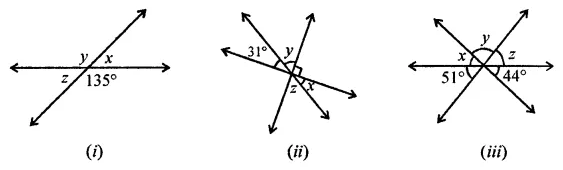Solution:Question 13.
In the given figure, lines AB and CD intersect at F. If ∠EFA = ∠AFD and ∠CFB = 50°, find ∠EFC.Solution: